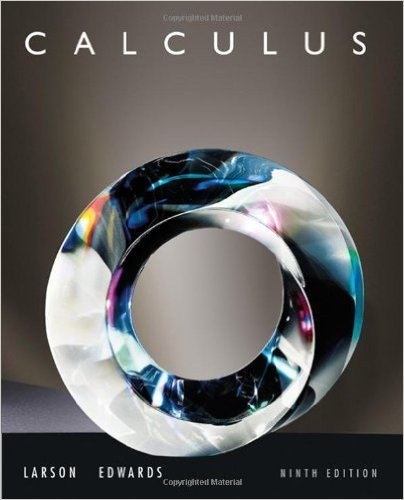×
×

# Solutions for Chapter 10.4: Polar Coordinates and Polar Graphs## Full solutions for Calculus | 9th Edition

ISBN: 9780547167022Solutions for Chapter 10.4: Polar Coordinates and Polar Graphs

Solutions for Chapter 10.4
4 5 0 285 Reviews
20
4
##### ISBN: 9780547167022

This expansive textbook survival guide covers the following chapters and their solutions. This textbook survival guide was created for the textbook: Calculus , edition: 9. Since 122 problems in chapter 10.4: Polar Coordinates and Polar Graphs have been answered, more than 61052 students have viewed full step-by-step solutions from this chapter. Calculus was written by and is associated to the ISBN: 9780547167022. Chapter 10.4: Polar Coordinates and Polar Graphs includes 122 full step-by-step solutions.

Key Calculus Terms and definitions covered in this textbook
• Arc length formula

The length of an arc in a circle of radius r intercepted by a central angle of u radians is s = r u.

• Bar chart

A rectangular graphical display of categorical data.

• Coefficient matrix

A matrix whose elements are the coefficients in a system of linear equations

• Cofunction identity

An identity that relates the sine, secant, or tangent to the cosine, cosecant, or cotangent, respectively

• Coordinate(s) of a point

The number associated with a point on a number line, or the ordered pair associated with a point in the Cartesian coordinate plane, or the ordered triple associated with a point in the Cartesian three-dimensional space

• Equal complex numbers

Complex numbers whose real parts are equal and whose imaginary parts are equal.

• Exponential growth function

Growth modeled by ƒ(x) = a ? b a > 0, b > 1 .

• Graph of an inequality in x and y

The set of all points in the coordinate plane corresponding to the solutions x, y of the inequality.

• Index of summation

See Summation notation.

• Inverse reflection principle

If the graph of a relation is reflected across the line y = x , the graph of the inverse relation results.

• Limit at infinity

limx: qƒ1x2 = L means that ƒ1x2 gets arbitrarily close to L as x gets arbitrarily large; lim x:- q ƒ1x2 means that gets arbitrarily close to L as gets arbitrarily large

• Multiplication property of equality

If u = v and w = z, then uw = vz

• Probability function

A function P that assigns a real number to each outcome O in a sample space satisfying: 0 … P1O2 … 1, P12 = 0, and the sum of the probabilities of all outcomes is 1.

• Rational zeros

Zeros of a function that are rational numbers.

• Reciprocal function

The function ƒ(x) = 1x

• Remainder theorem

If a polynomial f(x) is divided by x - c , the remainder is ƒ(c)

• Solve a system

To find all solutions of a system.

A graph in which (-x, -y) is on the the graph whenever (x, y) is; or a graph in which (-r, ?) or (r, ? + ?) is on the graph whenever (r, ?) is

• Translation

See Horizontal translation, Vertical translation.

• Vertical translation

A shift of a graph up or down.

×